# CSS 技巧一则 -- 在 CSS 中使用三角函数绘制曲线图形及展示动画

## 函数: 第一步

 x= -2 -1 0 1 2 ... y= 4 1 0 1 4 ...图像会倒过来变成像 n 这样的样子？

就这样，我们可以通过这个函数，得到两种曲线，正的u 和 反的n那么问题来了，要画任意曲线，那么意味着，曲线要可大可小，可以在图中的任意一个位置，要怎么办呢？

f(x) = x^2 / -2

 x= -2 -1 0 1 2 ... y= -2 -1/2 0 -1 /2 -2 ...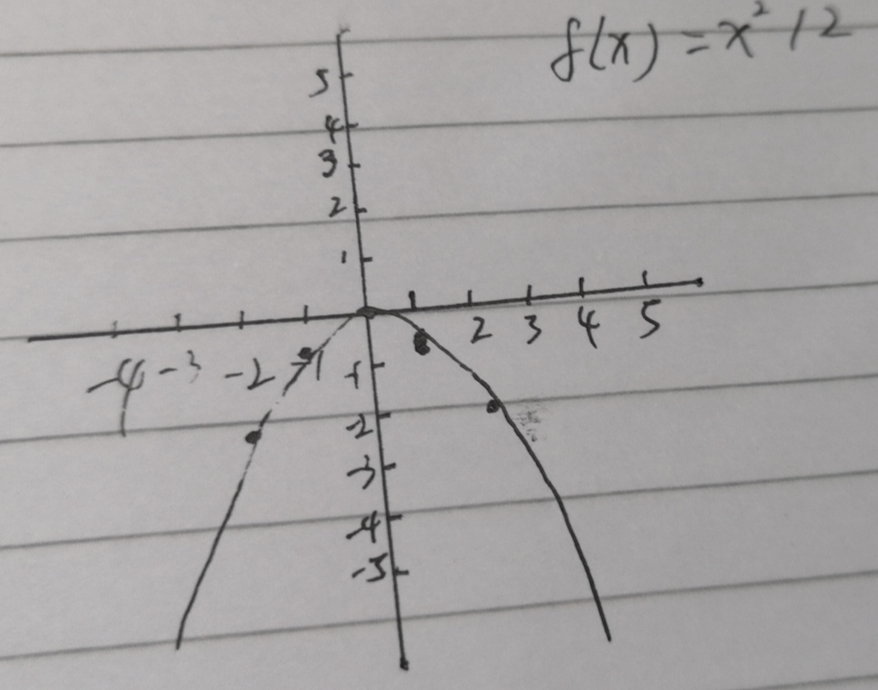y会因为除以2变得更小（想象一下两侧的y值会变小），当x = 2 ,  y就会等于2, 这样的结果是曲线变宽。

 x= -2 - 1 -1 - 1 0 - 1 1 - 1 2 - 1 3 - 1 y= 9 4 1 0 1 4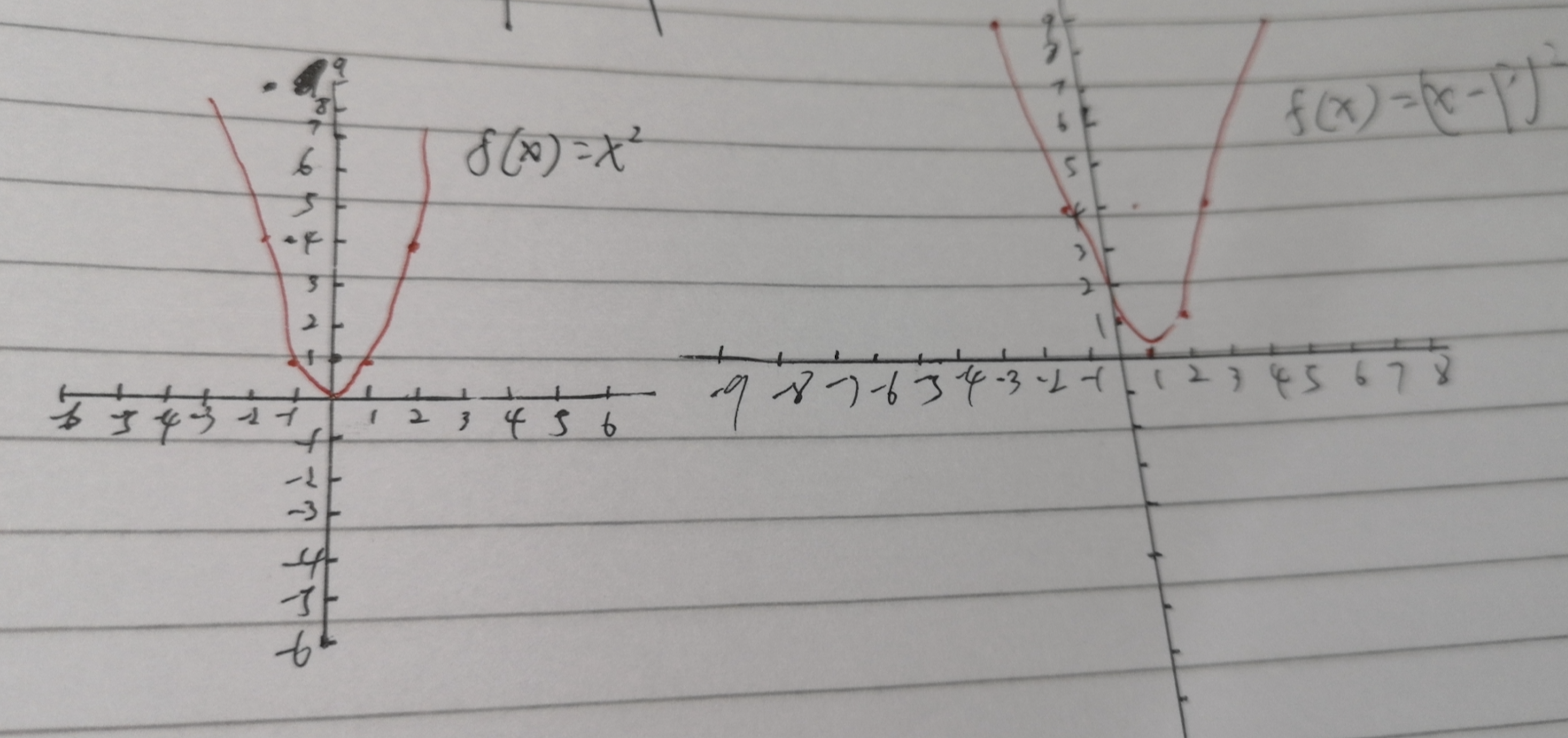x= -2 -1 0 1 2 ... y= 5 2 1 2 4 ...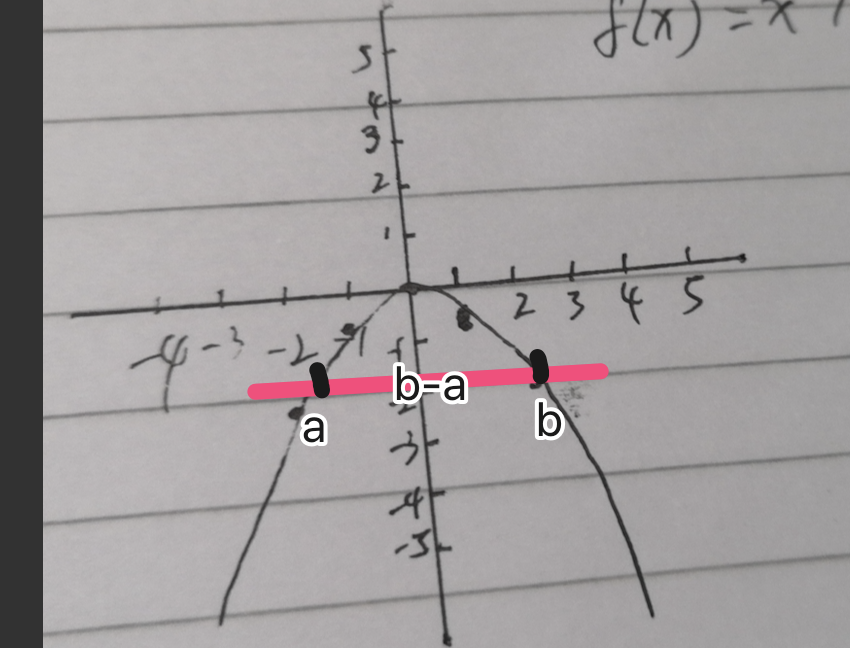## 接下来，工程问题，曲线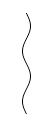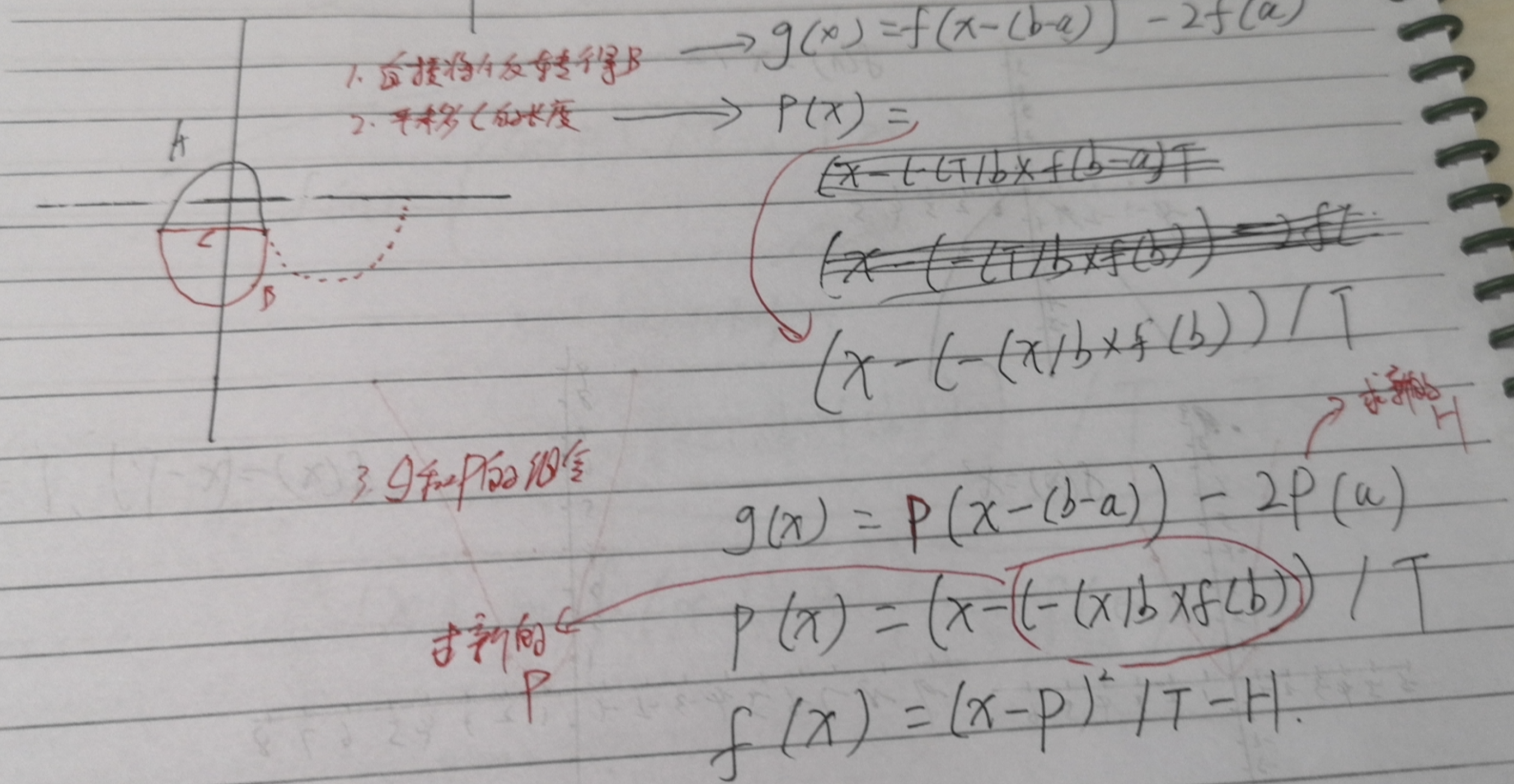// g(x) = f(x-(b-a)) - 2* f(a)， T < 0
function g (x, T, P, range) {
const [a, b] = range
return f(x - (b - a), T, P, range) - 2 * f(0, T, P, range)
}

// 当 T < 0 相当于上面图中的 p(x) = (x - (- (T / B * f(b - a))))  / T， T < 0
// 当 T > 0 直接计算 f(x) = (x - P) ^ 2 / T, T > 0
function f (x, T, P, range, s) {
const [a, b] = range
if (T < 0 && !s) {
return Math.pow(x - (-(T / b * f(b - a, T, P, range, true))), 2) / T
}
if (T > 0 || s) {
return Math.pow(x - P, 2) / T
}

}
// 选择初始函数
function getY (x, T, P, range) {
if (T > 0) {
return f(x, T, P, range)
} else {
return g(x, T, P, range)
}
}
//获取一堆x，y点组成的集合， size = 波浪数量，origin=原点，item = 配置P H T变量，points和ysize为递归存储数据
function GetPoints(size, origin, item, points = [], ysize) {
if (ysize === undefined) {
ysize = size
}
if (size <= 0) {
return points
}
const z = size % 2 === 0
const M = 1 // 密度
const width = item.b - item.a // 宽度
let i = width;
while (i >= -width) {
const point = [
(origin + i) + (ysize - size) * (width * 2), // x
origin + getY(i, (z ? item.T : -item.T), item.P, [ // y
item.a,
item.b
])
]
points.push(point)
i -= M;
}
GetPoints(size-1, origin, {
a: item.a,
b: item.b,
T: item.T,
P: item.P
}, points, ysize)
return points;
}

## 效果拉到本地跑一跑：

<!DOCTYPE html>
<html lang="en">
<meta charset="UTF-8">
<meta name="viewport" content="width=device-width, initial-scale=1.0">
<meta http-equiv="X-UA-Compatible" content="ie=edge">
<title>Document</title>
<style>
.circle {
position: absolute;
width: 1px;
height: 1px;
background: #333;
left: 0px;
top: 0px;
transition: all 200ms;
}
</style>
<body>
<div class="circle" id="circle"></div>
<script>
function g (x, T, P, range) {
const [a, b] = range
return f(x - (b - a), T, P, range) - 2 * f(0, T, P, range)
}

function f (x, T, P, range, s) {
const [a, b] = range
if (T < 0 && !s) {
return Math.pow(x - (-(T / b * f(b - a, T, P, range, true))), 2) / T
}
if (T > 0 || s) {
return Math.pow(x - P, 2) / T
}

}

function getY (x, T, P, range) {
if (T > 0) {
return f(x, T, P, range)
} else {
return g(x, T, P, range)
}
}
function GetPoints(size, origin, item, points = [], ysize) {
if (ysize === undefined) {
ysize = size
}
if (size <= 0) {
return points
}
const z = size % 2 === 0
const M = 1 // 密度
const width = item.b - item.a // 宽度
let i = width;
while (i >= -width) {
const point = [
(origin + i) + (ysize - size) * (width * 2), // x
origin + getY(i, (z ? item.T : -item.T), item.P, [ // y
item.a,
item.b
])
]
points.push(point)
i -= M;
}
GetPoints(size-1, origin, {
a: item.a,
b: item.b,
T: item.T,
P: item.P
}, points, ysize)
return points;
}

/**
*/
function getBoxShadow (color = '#333') {
let points = GetPoints(6, [500, 100], {
a : 0,
b : 100,
T : 200,
P : 0
})

// const s = []
const s = points.map((point) => ${point}px${point}px 0 0 ${color}) return s.join(',') } document.querySelector('#circle').style.cssText = box-shadow:${getBoxShadow()}; transform: rotate(90deg) translate(-500px, -500px)

</script>
</body>
</html>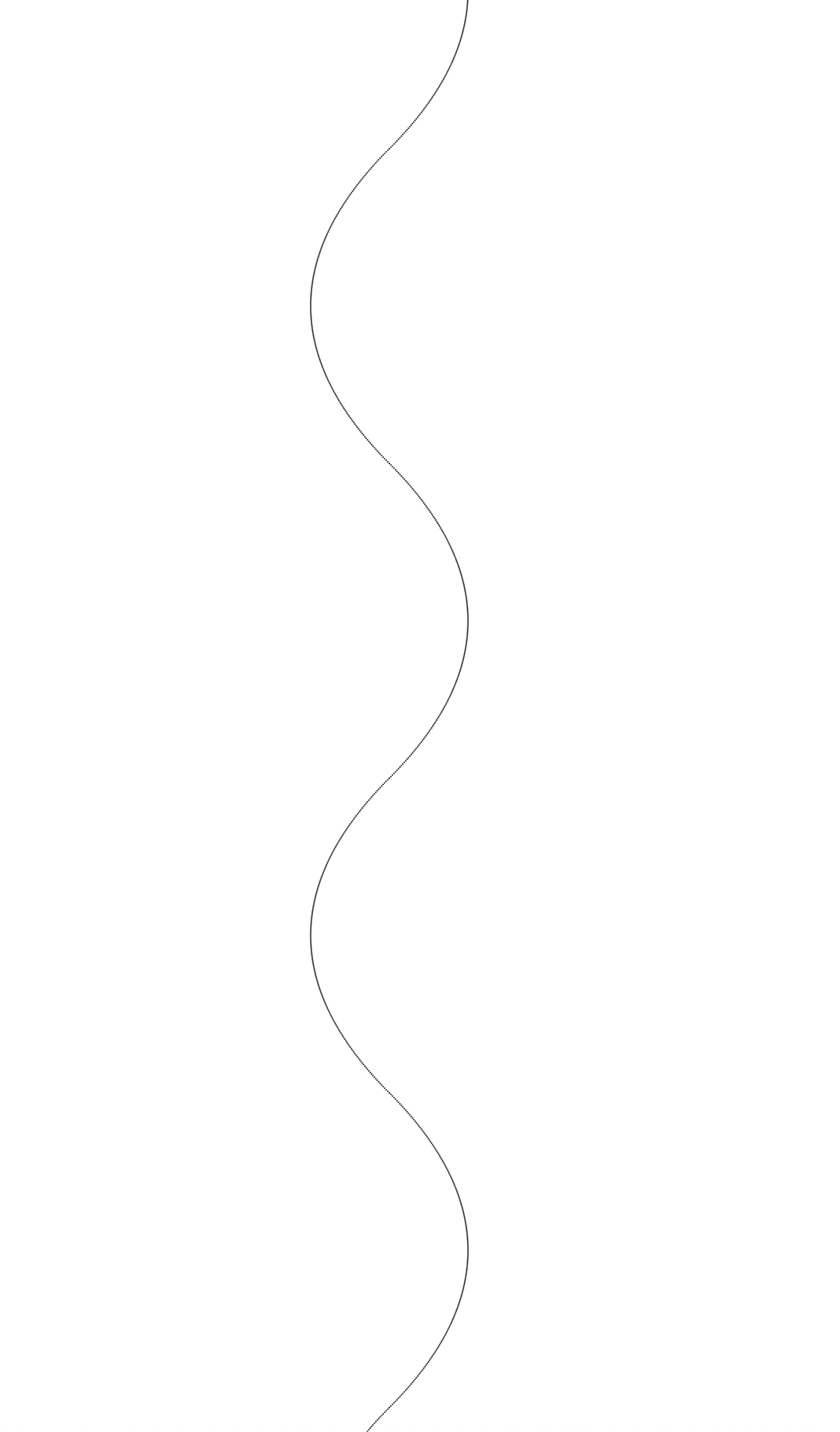## 展望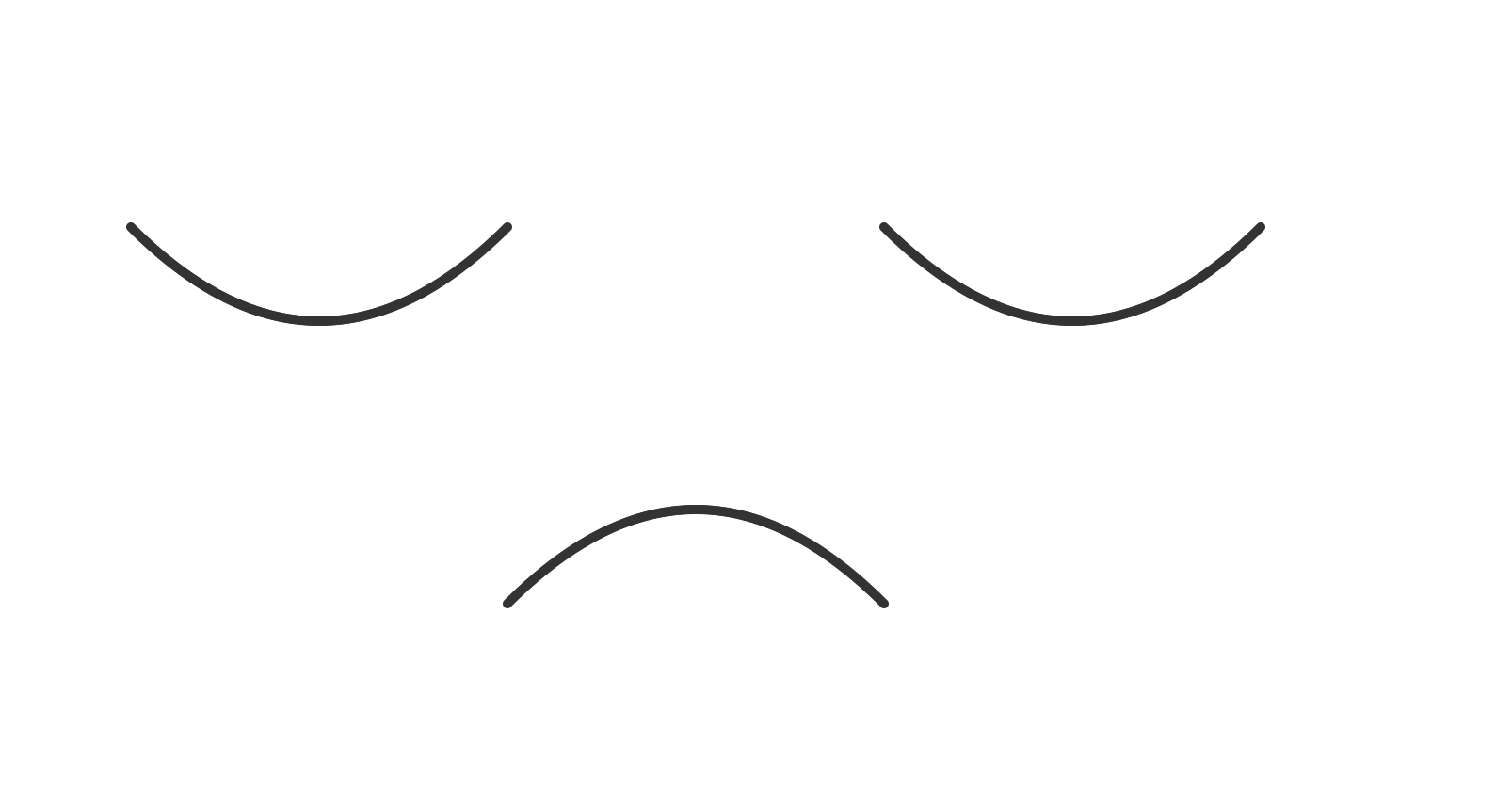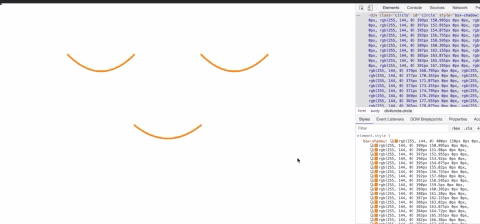## 延续

posted @ 2019-12-01 20:43  张泰峰  阅读(438)  评论(0编辑  收藏